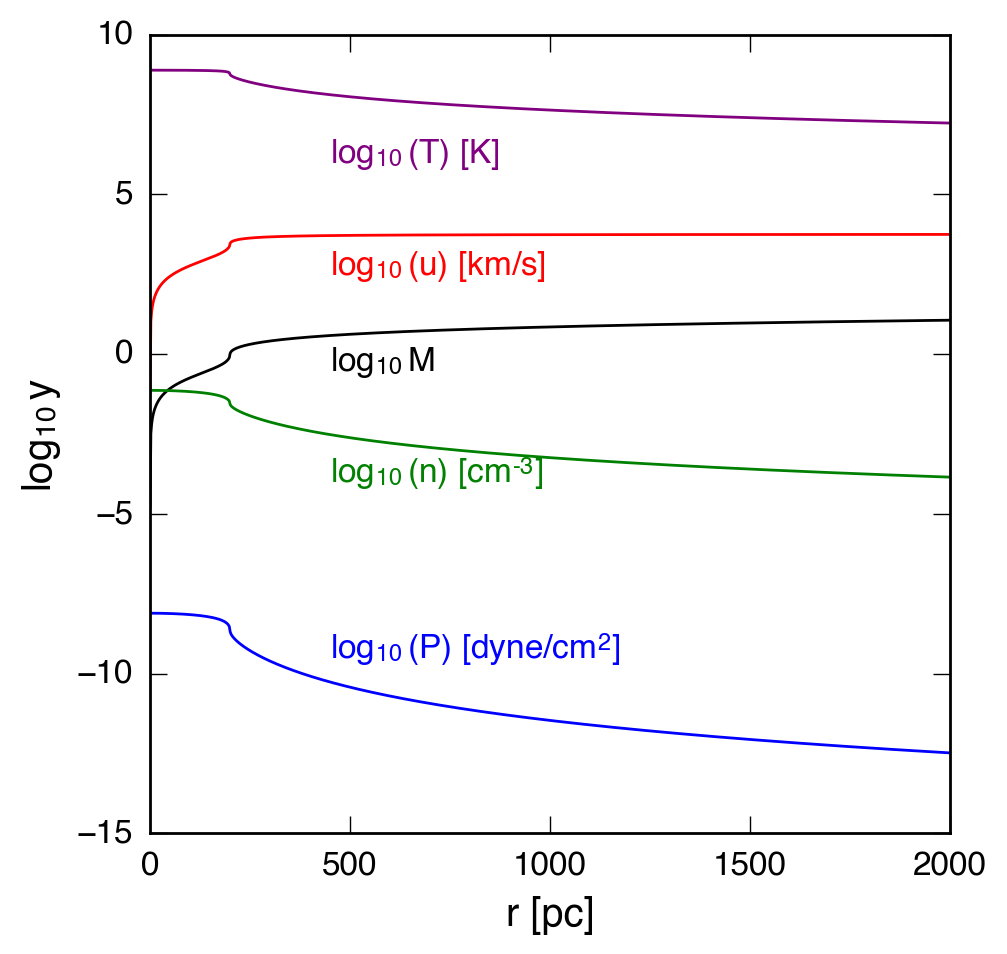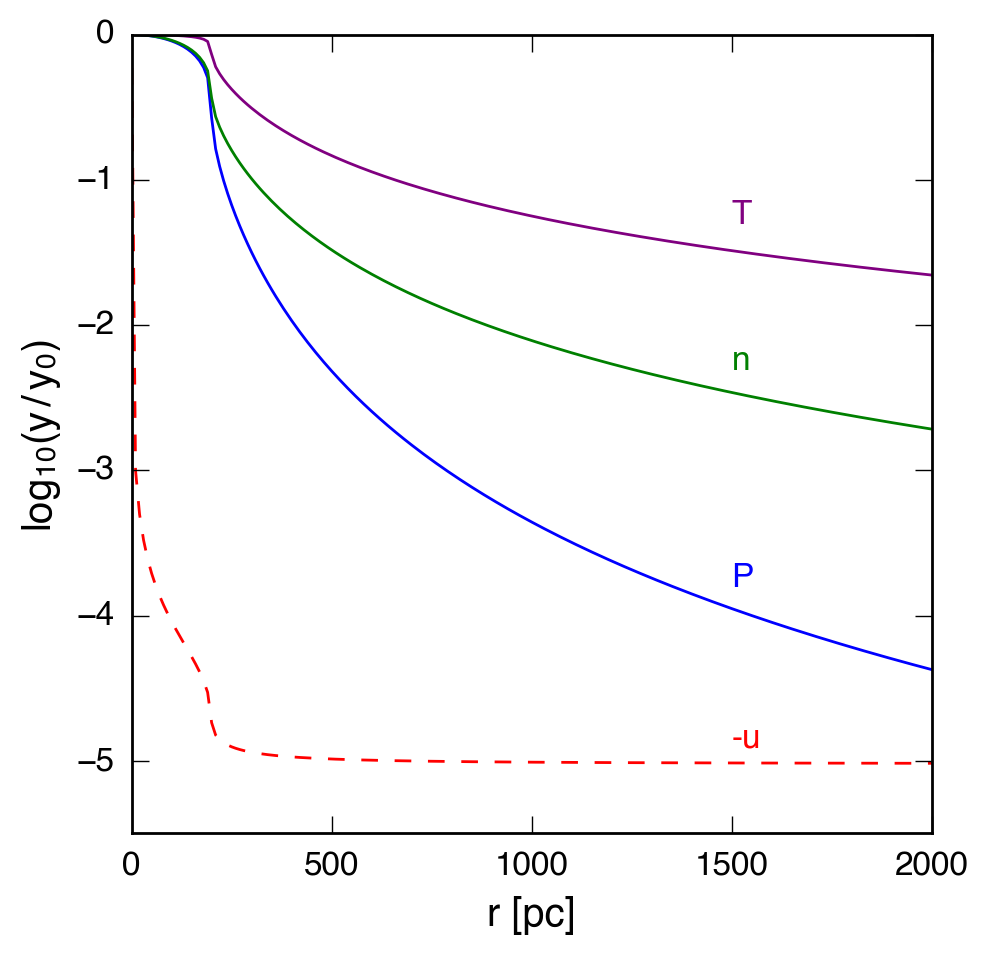Chevalier & Clegg Wind Model

January 8, 2016

We need to come up with a reasonable set of parameter space to explore in studying the environment of the cool material in galactic winds. The Chevalier and Clegg wind model (1985) provides a reasonable fit for the escaping hot wind, which can be sampled at a variety of radii from the galaxy to give a reasonable set of densities, veloicties, and temperatures.

The CC85 model with default parameters is shown below:And a version with each variable normalized to the value at $r = 0$:These parameters include a driving region of size $R = 200$ pc, mass input rate $\dot{M} = 1.0 \, \mathrm{M}_{\odot} \, \mathrm{yr}^{-1}$, and energy input rate $\dot{E} = 10^{43} \, \mathrm{erg} \, \mathrm{s}^{-1}$.